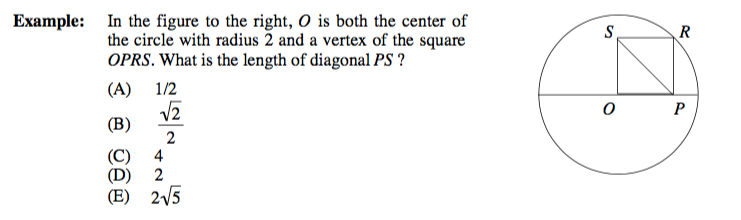top of page
Search
• Yuzhe

# Maths skill in GMAT/GRE

Most geometry problems on the test require straightforward calculations. However, some problems measure your insight into the basic rules of geometry. For this type of problem, you should step back and take a “birds-eye” view of the problem. The following example will illustrate.The diagonals of a square are equal. Hence, line segment OR (not shown) is equal to SP. Now, OR is a radius of the circle and therefore OR = 2. Hence, SP = 2 as well, and the answer is (D).

bottom of page